## Thursday, April 20, 2017

### Extracting the positions of character state changes from stochastic map trees in phytools

Yesterday, a friend & colleague emailed me the following question:

“Me gustaría preguntarte si se pueden extraer las fechas estimadas de las transiciones de estado en un análisis SIMMAP en phytools?”

that is:

“I'd like to ask you if one can extract the estimated dates of state transition in a stochastic mapping (SIMMAP) analysis in phytools?”

The answer is “yes” - using the function `markChanges`. For instance:

``````library(phytools)
tree
``````
``````##
## Phylogenetic tree with 26 tips and 25 internal nodes.
##
## Tip labels:
##  A, B, C, D, E, F, ...
##
## Rooted; includes branch lengths.
``````
``````x
``````
``````## A B C D E F G H I J K L M N O P Q R S T U V W X Y Z
## b b a a b a b b a a a a a a a a b b b b b b b a a b
## Levels: a b
``````
``````mtree<-make.simmap(tree,x)
``````
``````## make.simmap is sampling character histories conditioned on the transition matrix
##
## Q =
##            a          b
## a -0.9111912  0.9111912
## b  0.9111912 -0.9111912
## (estimated using likelihood);
## and (mean) root node prior probabilities
## pi =
##   a   b
## 0.5 0.5
``````
``````## Done.
``````
``````cols<-setNames(c("blue","red"),c("a","b"))
plot(mtree,cols,ftype="i",lwd=3,mar=c(4.1,1.1,1.1,1.1))
axis(1)
obj<-markChanges(mtree,colors=cols,lwd=3)
``````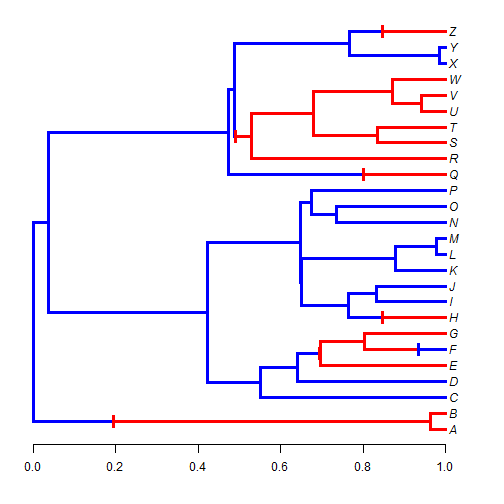Here, I've marked the sampled changes on the tree - but I've also extracted their positions above the root in the matrix `obj`:

``````obj
``````
``````##              x       y
## a->b 0.1955114  1.5000
## a->b 0.6950576  5.7500
## b->a 0.9342177  6.0000
## a->b 0.8472460  8.0000
## a->b 0.8019033 17.0000
## a->b 0.4896849 19.4375
## a->b 0.8475341 26.0000
``````

We can see this if we drop our marked changes to the x-axis:

``````plot(mtree,cols,ftype="i",lwd=3,mar=c(4.1,1.1,1.1,1.1))
axis(1)
obj<-markChanges(mtree,plot=FALSE)
for(i in 1:nrow(obj)) lines(rep(obj[i,1],2),c(0,obj[i,2]),lty="dotted",
col=make.transparent("grey",0.8))
points(obj[,1],rep(0.2,nrow(obj)),pch=21,bg="grey")
markChanges(mtree,cols,lwd=3)
``````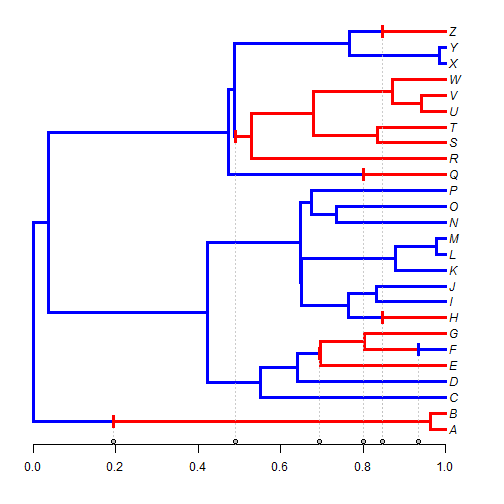Normally, however, we should probably obtain a set of trees from the posterior distribution - rather than a single tree. For instance:

``````mtrees<-make.simmap(tree,x,nsim=100)
``````
``````## make.simmap is sampling character histories conditioned on the transition matrix
##
## Q =
##            a          b
## a -0.9111912  0.9111912
## b  0.9111912 -0.9111912
## (estimated using likelihood);
## and (mean) root node prior probabilities
## pi =
##   a   b
## 0.5 0.5
``````
``````## Done.
``````

Just a reminder that phytools already includes a cool S3 `density` function for the number of changes of different types in an object of class `"multiSimmap"` consisting of a posterior sample of trees:

``````obj<-density(mtrees)
obj
``````
``````##
## Distribution of changes from stochastic mapping:
##  a->b        b->a
##  Min.   :1   Min.   :1
##  Median :5   Median :3
##  Mean   :4.81    Mean   :3.46
##  Max.   :9   Max.   :7
##
## 95% HPD interval(a->b): [1, 6]
## 95% HPD interval(b->a): [1, 6]
``````
``````plot(obj)
``````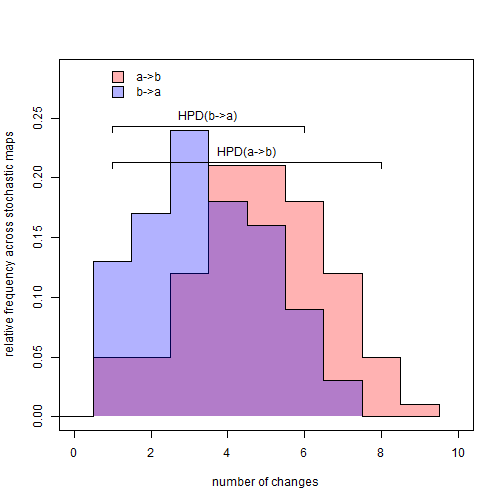We can also try to get a posterior density on the positions of these various changes between states:

``````dotTree(tree,x,colors=cols,ftype="i",lwd=3,mar=c(4.1,1.1,1.1,1.1))
axis(1)
obj<-sapply(mtrees,markChanges,colors=sapply(cols,make.transparent,alpha=0.1))
add.simmap.legend(colors=sapply(setNames(cols[2:1],c("a->b","b->a")),
make.transparent,0.4),prompt=FALSE,x=0,y=26,vertical=TRUE)
``````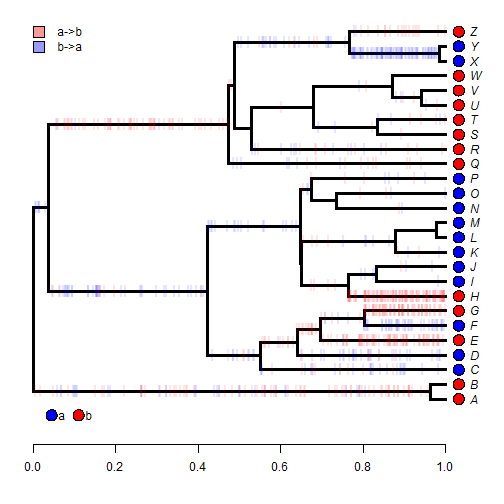This is just a list of matrices. For instance, here are the first 10:

``````obj[1:10]
``````
``````## []
##              x        y
## b->a 0.2636368  8.34375
## a->b 0.7965588  5.00000
## a->b 0.9748351  7.00000
## a->b 0.9750953  8.00000
## b->a 0.3070973 19.67188
## a->b 0.4710849 19.67188
## b->a 0.9217101 24.50000
##
## []
##               x        y
## b->a 0.08406267  8.34375
## a->b 0.70315021  5.00000
## a->b 0.89604958  7.00000
## a->b 0.76897714  8.00000
## b->a 0.67039182 25.25000
## a->b 0.68162989 25.25000
## b->a 0.96638624 24.50000
##
## []
##               x        y
## a->b 0.37282213  1.50000
## b->a 0.61444432  1.50000
## a->b 0.88630420  1.50000
## a->b 0.87318915  3.00000
## b->a 0.94439736  3.00000
## a->b 0.89377892  5.00000
## a->b 0.81464365  7.00000
## a->b 0.91710938  8.00000
## a->b 0.07535913 19.67188
## b->a 0.89555680 24.50000
##
## []
##              x        y
## b->a 0.2229673  8.34375
## a->b 0.7156098  5.00000
## a->b 0.9524018  7.00000
## a->b 0.8485678  8.00000
## b->a 0.8271941 24.50000
##
## []
##              x        y
## a->b 0.7918600  1.50000
## a->b 0.8387755  5.00000
## a->b 0.9637919  7.00000
## a->b 0.8172940  8.00000
## a->b 0.6557096 15.25000
## b->a 0.6655496 15.25000
## a->b 0.2856692 19.67188
## b->a 0.8764252 24.50000
##
## []
##              x        y
## a->b 0.5567777  1.50000
## a->b 0.9484923  5.00000
## a->b 0.9339515  7.00000
## a->b 0.8220921  8.00000
## a->b 0.2525068 19.67188
## b->a 0.7149046 25.25000
## a->b 0.9215291 26.00000
##
## []
##              x        y
## a->b 0.3328872  1.50000
## a->b 0.6776737  5.75000
## b->a 0.7405165  6.50000
## a->b 0.7941714  6.50000
## b->a 0.8458889  6.00000
## a->b 0.9726573  8.00000
## a->b 0.2351046 19.67188
## b->a 0.9340201 24.50000
##
## []
##               x        y
## a->b 0.45241952  1.50000
## a->b 0.04991146  8.34375
## b->a 0.15720353  8.34375
## a->b 0.67075616  5.75000
## b->a 0.86765865  6.00000
## a->b 0.99840460  8.00000
## a->b 0.29504460 19.67188
## b->a 0.71119438 25.25000
## a->b 0.92642095 26.00000
##
## []
##              x        y
## b->a 0.3217994  8.34375
## a->b 0.8831721  5.00000
## a->b 0.8332154  7.00000
## a->b 0.9316393  8.00000
## b->a 0.8609643 24.50000
##
## []
##              x        y
## b->a 0.3951734  1.50000
## a->b 0.8865010  1.50000
## b->a 0.4090050  8.34375
## a->b 0.9239062  5.00000
## a->b 0.9447590  7.00000
## a->b 0.9709664  8.00000
## b->a 0.6098279 25.25000
## a->b 0.6222670 25.25000
## b->a 0.9539116 24.50000
``````

It seems like we should first accumulate the x column of all these matrices into a single vector:

``````X<-unlist(sapply(obj,function(x) x[,1]))
``````

Now the changes of each type:

``````transitions<-sort(unique(names(X)))
heights<-lapply(transitions,function(n,x) x[which(names(x)==n)],x=X)
obj<-lapply(heights,hist,plot=FALSE)
par(mfrow=c(2,1))
barplot(obj[]\$counts/length(mtrees),space=0,cex.names=0.75,
names.arg=paste(obj[]\$breaks[1:10],obj[]\$breaks[2:11],sep="-"),
main=paste("transitions",names(heights[])),
ylab=paste("average number of changes",names(heights[])),
xlab="height above the root")
axis(1,at=1:10-0.5,labels=rep("",10))
barplot(obj[]\$counts/length(mtrees),space=0,cex.names=0.75,
names.arg=paste(obj[]\$breaks[1:10],obj[]\$breaks[2:11],sep="-"),
main=paste("transitions",names(heights[])),
ylab=paste("average number of changes",names(heights[])),
xlab="height above the root")
axis(1,at=1:10-0.5,labels=rep("",10))
``````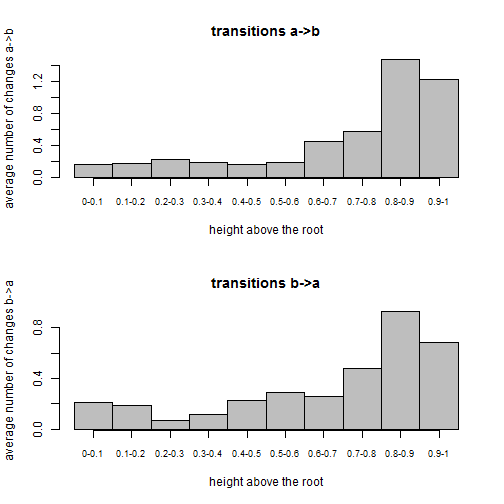Note that there is a lot more edge lengths towards the tips of the tree - which explains why we tend to see a lot more reconstructed changes in that region.

This example uses a binary character, but it could easily be extended (with some modifications, obviously) to a multistate character.

The data & tree were simulated as follows:

``````Q<-matrix(c(-1,1,1,-1),2,2)
rownames(Q)<-colnames(Q)<-letters[1:2]
x<-as.factor(sim.history(tree<-pbtree(n=26,tip.label=LETTERS,scale=1),Q)\$states)
``````

#### 4 comments:

1.Hi Liam,

Thanks for the great resource! I've been trying to extract the distribution of state changes following the code you've presented on this page but I get the following error message:

> obj<-density(mtrees)
Error in density.default(mtrees) : argument 'x' must be numeric

Everything works great up until that point. Any idea what my problem is? Here's the preceding code and output, if that makes a difference:

> mtrees<-make.simmap(tree2,states2,nsim=100)
make.simmap is sampling character histories conditioned on the transition matrix

Q =
1 2 3
1 -0.014773612 0.01228084 0.002492774
2 0.012280838 -0.02237385 0.010093012
3 0.002492774 0.01009301 -0.012585787
(estimated using likelihood);
and (mean) root node prior probabilities
pi =
1 2 3
0.3333333 0.3333333 0.3333333
Done.

1.What version of phytools are you using? You may need to update the package.

2.Updated to the latest version and that worked. Many thanks for your prompt reply and solution!

3.Hi Liam,

Thanks for the very clear explanation. A challenge with markChanges() is that, in some cases like BEAST dating analyses, the scale of each tree can be different as the root may vary over a few time units (eg. myr). Do you have thoughts on either rescaling trees prior to make.simmap() or a way to rescale "obj[x] based on the tree length it was estimated from? Thanks!

Note: due to the very large amount of spam, all comments are now automatically submitted for moderation.